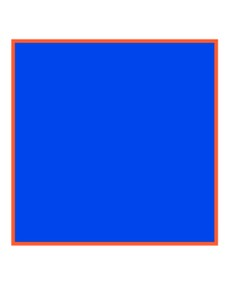Understanding what perimeter is

# Understanding what perimeter is

Students learn to understand what perimeter is.8,000 schools use Gynzy92,000 teachers use Gynzy1,600,000 students use Gynzy

## General

The students learn what perimeter is and when this happens.

## Standards

CCSS.Math.Content.3.MD.D.8

## Learning objective

Students will be able to tell what the concept of perimeter means (understanding).

## Introduction

On the interactive whiteboard there are two images. The students determine which is smaller in real life. The scooter is smaller than the car. After this you show three images. The students must state what the correct order is from small to large.

## Instruction

You first explain that the perimeter gives how long something is if you measure around it. Next you can have the students experience what perimeter is on their own by tracing an eraser and their hand to show their perimeter on a piece of paper. You explain that the line that they have drawn is the perimeter of the shape. After this you explain that the word perimeter means that you are measuring around something. You have the students determine which color the perimeter is for various shapes. Then you explain that when you use perimeter, that means you want to know what the lengths of all the sides of the shape are added together. For example, if you want to put a border with tiles around the edge of a swimming pool. The students determine if the statements about perimeter after this are true or false.

To check whether the students know what perimeter is and when you use it, you can ask the following questions:
- What color is the perimeter in this shape?
- Can you name an example of something that is a perimeter of something?
- What has the larger perimeter: a football field or a classroom?

## Quiz

The students test their understanding of the concept of perimeter through ten exercises. For some of them there is a shape given, and the students must indicate what color the perimeter is drawn in. The other exercises are true/false questions, in which a statement is made about the perimeter of something, and students must determine whether the statement is true or false.

## Closing

You discuss again with the students that it is important to know what the perimeter is, because that is how you know when you need it. Next you show a number of shapes on the interactive whiteboard and the students tell which color the perimeter is on each shape. After this you have the students work in pairs. They take turns indicating the perimeter of an object. Have them come up with a few objects on their own for which they can indicate the perimeter. Discuss the perimeter of several objects together as a class, for which the pairs of students have indicated the perimeter.

## Teaching tips

When students have difficulty with understanding the meaning of the concept of perimeter, you can emphasize that perimeter means that you measure around an object or shape.

!---+Instruction materials
Erasers, books, and rulers

### The online teaching platform for interactive whiteboards and displays in schools

• Save time building lessons

• Manage the classroom more efficiently

• Increase student engagement